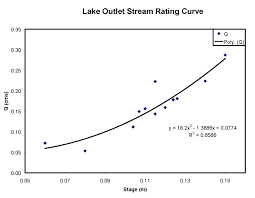## How to Calculate and Solve for Total Cross-Sectional Area of Gates | Design of Gating SystemThe image above represents total cross-sectional area of gates.

To compute for total cross-sectional area of gates, three essential parameters are needed and these parameters are Mass of the Casting (G), Specific Speed of Pouring (Ks) and Time of Pouring (t).

The formula for calculating total cross-sectional area of gates:

Fs = G / tKs

Where:

Fs = Total Cross-Sectional Area of Gates
G = Mass of the Casting
Ks = Specific Speed Of Pouring
t = Time of Pouring

Let’s solve an example;
Find the total cross-sectional area of gates when the mass of the casting is 10, specific speed of pouring is 18 and the time of pouring is 9.

This implies that;

G = Mass of the Casting = 10
Ks = Specific Speed Of Pouring = 18
t = Time of Pouring = 9

Fs = G / tKs
Fs = 10 / 9 x 18
Fs = 10 / 162
Fs = 0.0617

Therefore, the total cross-sectional area of gates is 0.0617 m2.

Calculating the Mass of the Casting when the Total Cross-Sectional Area of Gates, Specific Speed of Pouring and the Time of Pouring is Given.

G = Fs x tKs

Where:

G = Mass of the Casting
Fs = Total Cross-Sectional Area of Gates
Ks = Specific Speed Of Pouring
t = Time of Pouring

Let’s solve an example;
Find the mass of the casting when the total cross-sectional area of gates is 15, the specific speed of pouring is 7 and the time of pouring is 5.

This implies that;

Fs = Total Cross-Sectional Area of Gates = 15
Ks = Specific Speed Of Pouring = 7
t = Time of Pouring = 5

G = Fs x tKs
G = 15 x 5(7)
G = 15 x 35
G = 525

Therefore, the mass of the casting is 525 m.